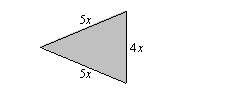Name:    Quiz 3.3-3.5

Multiple Choice
Identify the choice that best completes the statement or answers the question.

Write the word sentence as an equation. Then solve.

1.

19 more than a number c is 4.
 a.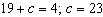c.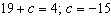b.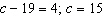d.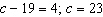2.

5 less than a number n is –1.
 a.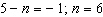c.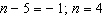b.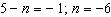d.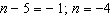3.

A number divided by –4 is –13.
 a.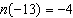; n = 52 c.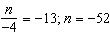b.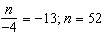d.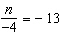; n =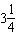4.

A number multiplied by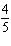is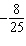.
 a.n =; n =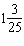c.n =; n =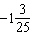b.n =; n =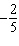d.n =; n =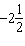Solve the equation. Check your solution.

5.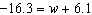a. 2.67 c. –10.2 b. -22.4 d. 10.2

6.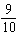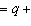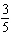a.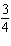c.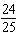b.d.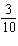7.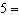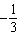c
 a.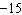c.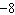b.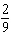d.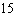8.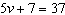a. 25 c. 35 b. 30 d. 6

Numeric Response

1.

The perimeter of the parallelogram is 30 feet. Find m.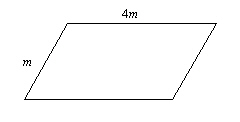Write an equation. Then solve.

1.

The temperature of dry ice is –109.6°F. This is 184.3°F less than the outside temperature. Write and solve an equation to find the outside temperature.

2.

You make \$0.46 for every newspaper you sell. A pair of soccer cleats costs \$92.92. How many newspapers do you have to sell to buy the soccer cleats?

3.

It cost \$2.75 to rent bowling shoes. Each game costs \$6.00. You have \$14.75. How many games can you bowl?

Write and solve an equation to find the unknown side length.

4.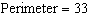cm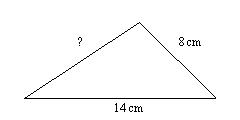Write and solve an equation to find x.

5.

Perimeter = 36 inches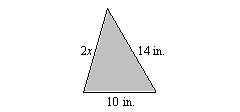6.

Perimeter = 126 yards